# Force_Lesson_Ch4.pdf

6 Mar 2023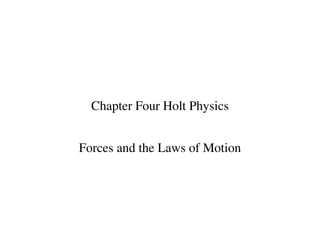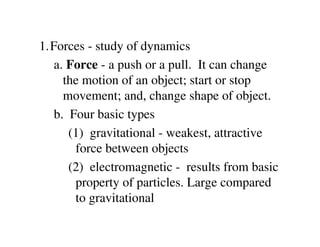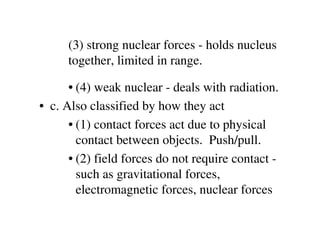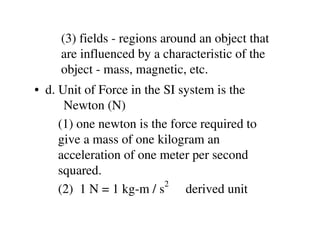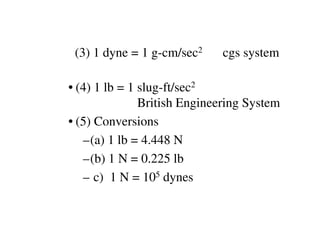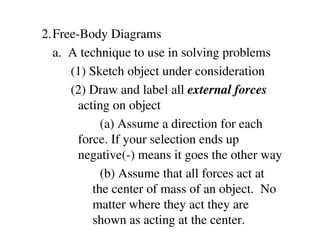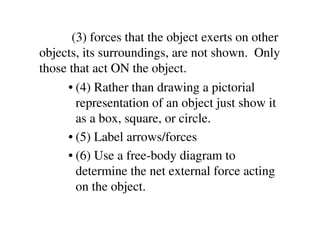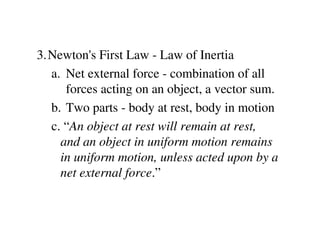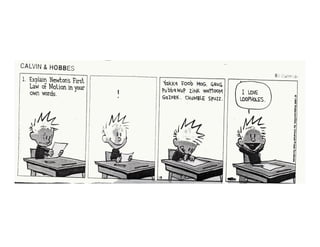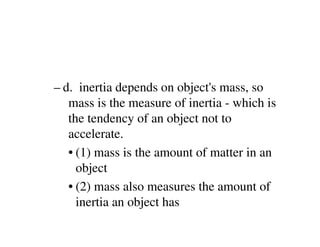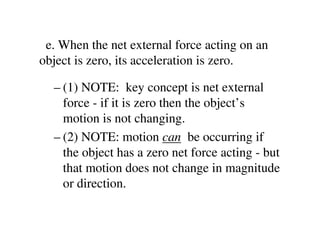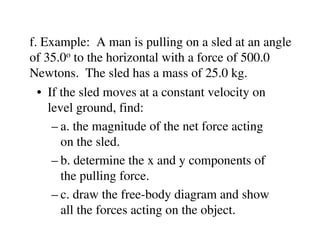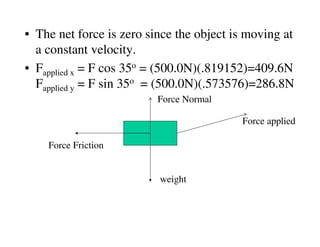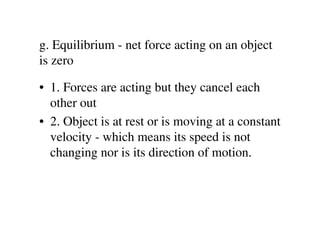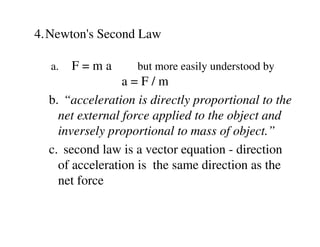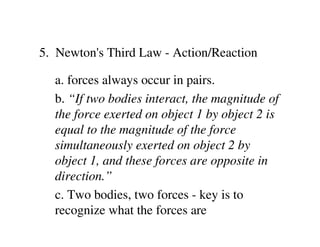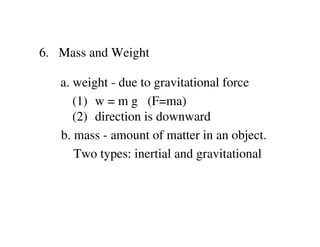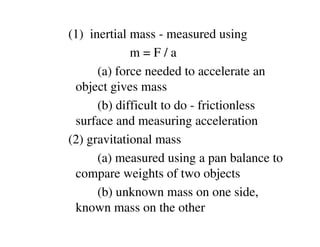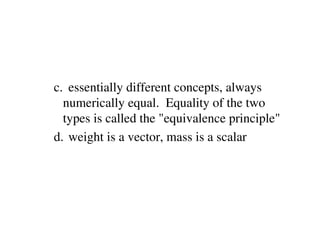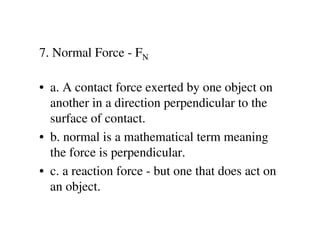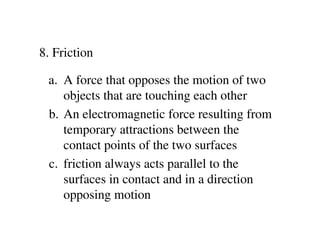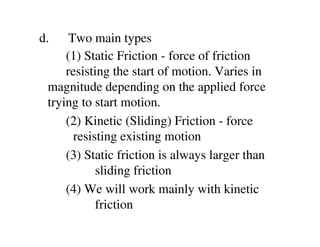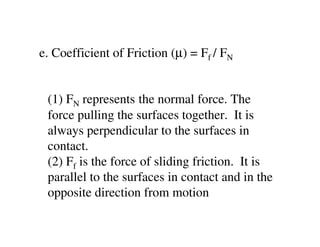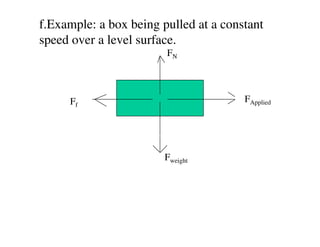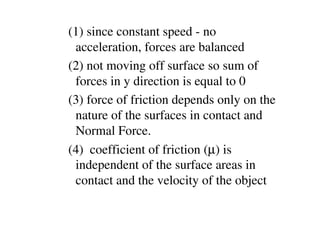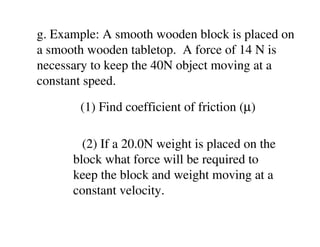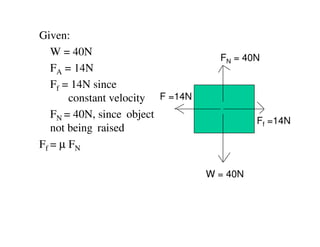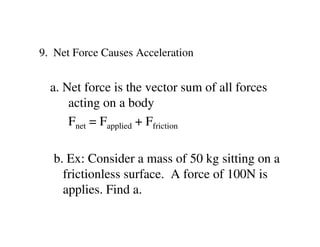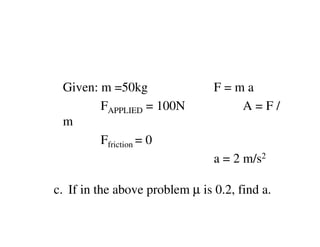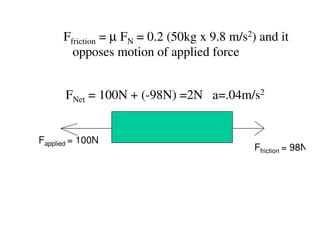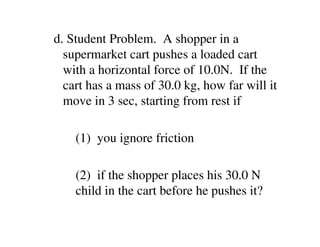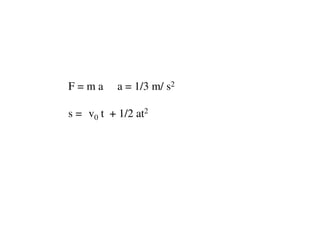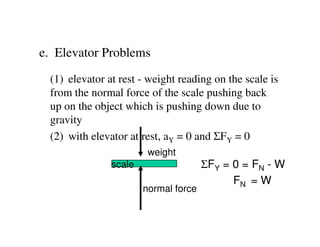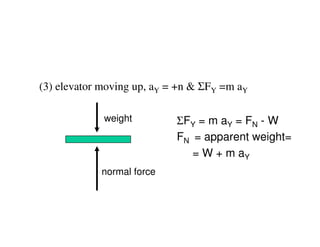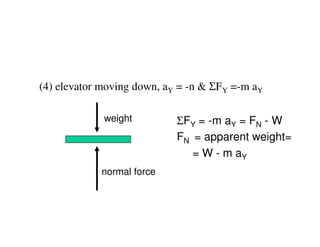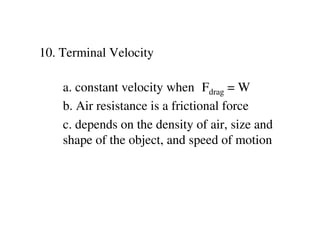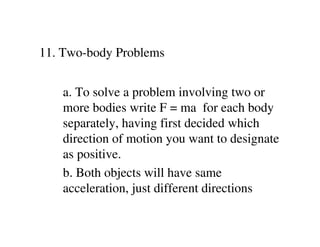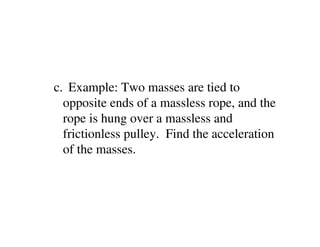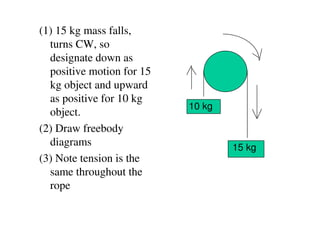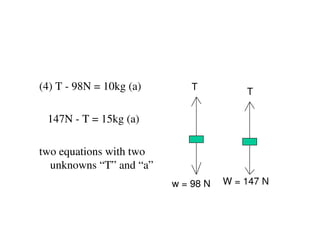1 sur 40

### Force_Lesson_Ch4.pdf

• 1. Chapter Four Holt Physics Forces and the Laws of Motion
• 2. 1.Forces - study of dynamics a. Force - a push or a pull. It can change the motion of an object; start or stop movement; and, change shape of object. b. Four basic types (1) gravitational - weakest, attractive force between objects (2) electromagnetic - results from basic property of particles. Large compared to gravitational
• 3. (3) strong nuclear forces - holds nucleus together, limited in range. • (4) weak nuclear - deals with radiation. • c. Also classified by how they act • (1) contact forces act due to physical contact between objects. Push/pull. • (2) field forces do not require contact - such as gravitational forces, electromagnetic forces, nuclear forces
• 4. (3) fields - regions around an object that are influenced by a characteristic of the object - mass, magnetic, etc. • d. Unit of Force in the SI system is the Newton (N) (1) one newton is the force required to give a mass of one kilogram an acceleration of one meter per second squared. (2) 1 N = 1 kg-m / s2 derived unit
• 5. (3) 1 dyne = 1 g-cm/sec2 cgs system • (4) 1 lb = 1 slug-ft/sec2 British Engineering System • (5) Conversions –(a) 1 lb = 4.448 N –(b) 1 N = 0.225 lb – c) 1 N = 105 dynes
• 6. 2.Free-Body Diagrams a. A technique to use in solving problems (1) Sketch object under consideration (2) Draw and label all external forces acting on object (a) Assume a direction for each force. If your selection ends up negative(-) means it goes the other way (b) Assume that all forces act at the center of mass of an object. No matter where they act they are shown as acting at the center.
• 7. (3) forces that the object exerts on other objects, its surroundings, are not shown. Only those that act ON the object. • (4) Rather than drawing a pictorial representation of an object just show it as a box, square, or circle. • (5) Label arrows/forces • (6) Use a free-body diagram to determine the net external force acting on the object.
• 8. 3.Newton's First Law - Law of Inertia a. Net external force - combination of all forces acting on an object, a vector sum. b. Two parts - body at rest, body in motion c. “An object at rest will remain at rest, and an object in uniform motion remains in uniform motion, unless acted upon by a net external force.”
• 10. – d. inertia depends on object's mass, so mass is the measure of inertia - which is the tendency of an object not to accelerate. • (1) mass is the amount of matter in an object • (2) mass also measures the amount of inertia an object has
• 11. e. When the net external force acting on an object is zero, its acceleration is zero. – (1) NOTE: key concept is net external force - if it is zero then the object’s motion is not changing. – (2) NOTE: motion can be occurring if the object has a zero net force acting - but that motion does not change in magnitude or direction.
• 12. f. Example: A man is pulling on a sled at an angle of 35.0o to the horizontal with a force of 500.0 Newtons. The sled has a mass of 25.0 kg. • If the sled moves at a constant velocity on level ground, find: – a. the magnitude of the net force acting on the sled. – b. determine the x and y components of the pulling force. – c. draw the free-body diagram and show all the forces acting on the object.
• 13. • The net force is zero since the object is moving at a constant velocity. • Fapplied x = F cos 35o = (500.0N)(.819152)=409.6N Fapplied y = F sin 35o = (500.0N)(.573576)=286.8N weight Force applied Force Normal Force Friction
• 14. g. Equilibrium - net force acting on an object is zero • 1. Forces are acting but they cancel each other out • 2. Object is at rest or is moving at a constant velocity - which means its speed is not changing nor is its direction of motion.
• 15. 4.Newton's Second Law a. F = m a but more easily understood by a = F / m b. “acceleration is directly proportional to the net external force applied to the object and inversely proportional to mass of object.” c. second law is a vector equation - direction of acceleration is the same direction as the net force
• 16. 5. Newton's Third Law - Action/Reaction a. forces always occur in pairs. b. “If two bodies interact, the magnitude of the force exerted on object 1 by object 2 is equal to the magnitude of the force simultaneously exerted on object 2 by object 1, and these forces are opposite in direction.” c. Two bodies, two forces - key is to recognize what the forces are
• 17. 6. Mass and Weight a. weight - due to gravitational force (1) w = m g (F=ma) (2) direction is downward b. mass - amount of matter in an object. Two types: inertial and gravitational
• 18. (1) inertial mass - measured using m = F / a (a) force needed to accelerate an object gives mass (b) difficult to do - frictionless surface and measuring acceleration (2) gravitational mass (a) measured using a pan balance to compare weights of two objects (b) unknown mass on one side, known mass on the other
• 19. c. essentially different concepts, always numerically equal. Equality of the two types is called the "equivalence principle" d. weight is a vector, mass is a scalar
• 20. 7. Normal Force - FN • a. A contact force exerted by one object on another in a direction perpendicular to the surface of contact. • b. normal is a mathematical term meaning the force is perpendicular. • c. a reaction force - but one that does act on an object.
• 21. 8. Friction a. A force that opposes the motion of two objects that are touching each other b. An electromagnetic force resulting from temporary attractions between the contact points of the two surfaces c. friction always acts parallel to the surfaces in contact and in a direction opposing motion
• 22. d. Two main types (1) Static Friction - force of friction resisting the start of motion. Varies in magnitude depending on the applied force trying to start motion. (2) Kinetic (Sliding) Friction - force resisting existing motion (3) Static friction is always larger than sliding friction (4) We will work mainly with kinetic friction
• 23. e. Coefficient of Friction (µ) = Ff / FN (1) FN represents the normal force. The force pulling the surfaces together. It is always perpendicular to the surfaces in contact. (2) Ff is the force of sliding friction. It is parallel to the surfaces in contact and in the opposite direction from motion
• 24. f.Example: a box being pulled at a constant speed over a level surface. FN FApplied Fweight Ff
• 25. (1) since constant speed - no acceleration, forces are balanced (2) not moving off surface so sum of forces in y direction is equal to 0 (3) force of friction depends only on the nature of the surfaces in contact and Normal Force. (4) coefficient of friction (µ) is independent of the surface areas in contact and the velocity of the object
• 26. g. Example: A smooth wooden block is placed on a smooth wooden tabletop. A force of 14 N is necessary to keep the 40N object moving at a constant speed. (1) Find coefficient of friction (µ) (2) If a 20.0N weight is placed on the block what force will be required to keep the block and weight moving at a constant velocity.
• 27. Given: W = 40N FA = 14N Ff = 14N since constant velocity FN = 40N, since object not being raised Ff = µ FN FN = 40N Ff =14N W = 40N F =14N
• 28. 9. Net Force Causes Acceleration a. Net force is the vector sum of all forces acting on a body Fnet = Fapplied + Ffriction b. Ex: Consider a mass of 50 kg sitting on a frictionless surface. A force of 100N is applies. Find a.
• 29. Given: m =50kg F = m a FAPPLIED = 100N A = F / m Ffriction = 0 a = 2 m/s2 c. If in the above problem µ is 0.2, find a.
• 30. Ffriction = µ FN = 0.2 (50kg x 9.8 m/s2) and it opposes motion of applied force FNet = 100N + (-98N) =2N a=.04m/s2 Ffriction = 98N Fapplied = 100N
• 31. d. Student Problem. A shopper in a supermarket cart pushes a loaded cart with a horizontal force of 10.0N. If the cart has a mass of 30.0 kg, how far will it move in 3 sec, starting from rest if (1) you ignore friction (2) if the shopper places his 30.0 N child in the cart before he pushes it?
• 32. F = m a a = 1/3 m/ s2 s = v0 t + 1/2 at2
• 33. e. Elevator Problems (1) elevator at rest - weight reading on the scale is from the normal force of the scale pushing back up on the object which is pushing down due to gravity (2) with elevator at rest, aY = 0 and ΣFY = 0 scale weight normal force ΣFY = 0 = FN - W FN = W
• 34. (3) elevator moving up, aY = +n & ΣFY =m aY weight normal force ΣFY = m aY = FN - W FN = apparent weight= = W + m aY
• 35. (4) elevator moving down, aY = -n & ΣFY =-m aY weight normal force ΣFY = -m aY = FN - W FN = apparent weight= = W - m aY
• 36. 10. Terminal Velocity a. constant velocity when Fdrag = W b. Air resistance is a frictional force c. depends on the density of air, size and shape of the object, and speed of motion
• 37. 11. Two-body Problems a. To solve a problem involving two or more bodies write F = ma for each body separately, having first decided which direction of motion you want to designate as positive. b. Both objects will have same acceleration, just different directions
• 38. c. Example: Two masses are tied to opposite ends of a massless rope, and the rope is hung over a massless and frictionless pulley. Find the acceleration of the masses.
• 39. (1) 15 kg mass falls, turns CW, so designate down as positive motion for 15 kg object and upward as positive for 10 kg object. (2) Draw freebody diagrams (3) Note tension is the same throughout the rope 10 kg 15 kg
• 40. (4) T - 98N = 10kg (a) 147N - T = 15kg (a) two equations with two unknowns “T” and “a” T T w = 98 N W = 147 N